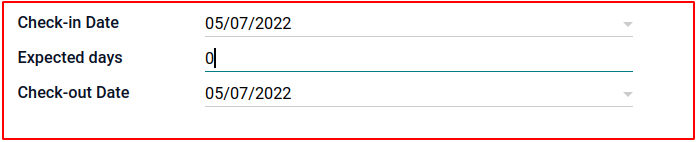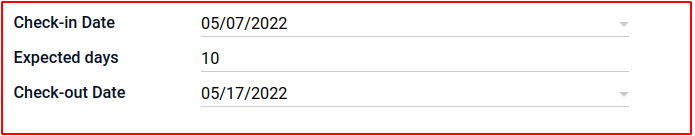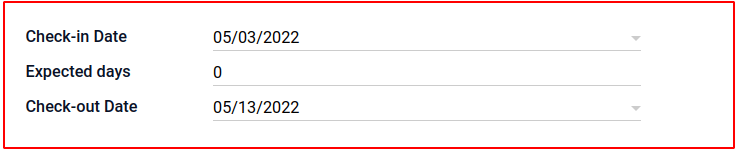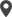Enable Dark Mode!By: Nila UJ

# How to Add Inverse Function for Compute Fields in Odoo 15

## Technical Odoo 15

The Odoo inverse method, as the name says, performs the inverse of the compute function: the invoked records have particular values for a field, to which you must apply the necessary changes on the field dependencies such that the computation gives us back the expected value.

By default, compute fields are read-only. Their primary purpose is to reverse this compute function. With the help of inverse, we can make the parameters of the field editable.

This blog explains how to add computed fields and inverse for computed fields to a model in Odoo.

1. Defining the computed function

2. Defining the Inverse function

Compute Function

What is a computed field?

A computed field is a field that can compute values related to other fields. It is also similar to other fields. Additionally, it has one argument compute. The name of the function starts with _compute_function_name. The value will be computed for this field automatically. The compute field value is non-editable. It means the value must be in read-only format.

For example hotel management, In hotel management, there will be a check-in date, check-out date, and expected days(planning days of the customer). Here check-out date will be computed field.

First of all, we have to define a compute field as shown below:

```check_out_date = fields.Date(string='Check-out Date',
default=fields.datetime.now(),
compute="_compute_check_out_date")```

Here, we have to give argument compute. In this argument, we have to pass the function name which is used for the calculation. In this case, our compute field is check_out_date. And the passed function is _compute_check_out_date.

Defining compute function

In this case, this function will take inputs like check-in date and expected days. Then the check-out date will be computed using this function.

Here the argument compute is given and now let’s define the '_compute_check_out_date' function definition should be like this:

```@api.depends('check_in_date')def _compute_check_out_date(self):   if self.check_in_date:       self.check_out_date =self.check_in_date +
timedelta(days=self.expected_days)```

This function calculates the expected check-out date; this is how computed fields in Odoo work.

OUTPUT

Initially, expected days are zero.Then we have to give expected days. As a result, the check-out Date is computed. The check-out Date is non-editableInverse Function

The inverse function is mainly used to make compute field editable. A user can’t change a compute field value. This problem can simply be solved with the help of an inverse parameter. It will make a compute field editable. The main purpose is to reverse the function. This inverse parameter can be given along with the compute parameter. The other purpose is for making reverse the functionality.

The inverse parameter will give along with the compute parameter. After the compute parameter, we have to specify the inverse parameter. This inverse parameter will pass an inverse function name. This inverse function should be specified as _inverse_function_name.

`check_out_date = fields.Date(string='Check-out Date',                            default=fields.datetime.now(),                           compute="_compute_check_out_date",                           inverse="_inverse_check_out_date")`

Here, the inverse parameter is used along with compute parameter. The inverse parameter passes the inverse function name.

Definition of simple Inverse function

In this case, the check-out date is non-editable. Hence using this function will help the user to edit the compute field.

`def _inverse_check_out_date(self):   pass`

OUTPUT

In this output, the check-out date is changed to an editable format. Now the user can change the compute field. This is a simple inverse function.Definition Inverse function

The other purpose of the inverse function is to make the reverse of the functionality. The workflow of function can reverse. In this case, select the check-out date and check-in date. Then the corresponding expected days will be computed. The inverse function will be shown below:

`@api.depends('check_in_date')def _compute_check_out_date(self):   if self.check_in_date:       self.check_out_date = self.check_in_date + timedelta(           days=self.expected_days)def _inverse_check_out_date(self):   if self.check_out_date:       self.expected_days = (self.check_out_date - self.check_in_date).days   pass`

In this case, expected days are calculated. Here check out date and check-in date should be given. Then the corresponding expected days will be calculated automatically.

OUTPUTThis is how the functioning of inverse functioning is.These are the primary purposes of an inverse function.

If you need any assistance in odoo, we are online, please chat with us.

### Recent Posts

#### How to Plan a Task in Odoo 16 Field ServicesCalicut

Cybrosys Technologies Pvt. Ltd.
Neospace, Kinfra Techno Park
Kakkancherry, Calicut
Kerala, India - 673635London

Cybrosys Limited
Alpha House,
100 Borough High Street, London,
SE1 1LB, United KingdomKochi

Cybrosys Technologies Pvt. Ltd.
1st Floor, Thapasya Building,
Kochi, India - 682030.Bangalore

Cybrosys Techno Solutions
The Estate, 8th Floor,